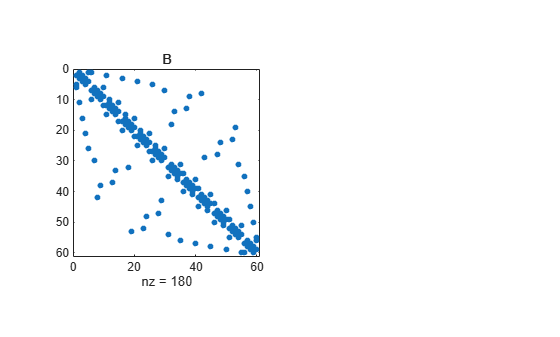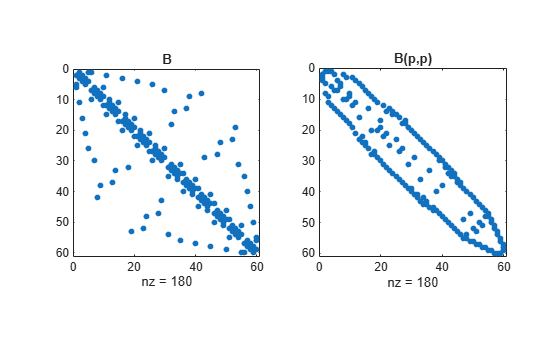symrcm

Sparse reverse Cuthill-McKee ordering

r = symrcm(S)

Description

r = symrcm(S) returns the symmetric reverse Cuthill-McKee ordering of S. This is a permutation r such that S(r,r) tends to have its nonzero elements closer to the diagonal. This is a good preordering for LU or Cholesky factorization of matrices that come from long, skinny problems. The ordering works for both symmetric and nonsymmetric S.

For a real, symmetric sparse matrix, S, the eigenvalues of S(r,r) are the same as those of S, but eig(S(r,r)) probably takes less time to compute than eig(S).

Examples

collapse all

The statement

B = bucky;

uses a function in the demos toolbox to generate the adjacency graph of a truncated icosahedron. This is better known as a soccer ball, a Buckminster Fuller geodesic dome (hence the name bucky), or, more recently, as a 60-atom carbon molecule. There are 60 vertices. The vertices have been ordered by numbering half of them from one hemisphere, pentagon by pentagon; then reflecting into the other hemisphere and gluing the two halves together.

With this numbering, the matrix does not have a particularly narrow bandwidth, as the first spy plot shows:

figure();
subplot(1,2,1),spy(B),title('B')The reverse Cuthill-McKee ordering is obtained with:

p = symrcm(B);
R = B(p,p);

The spy plot shows a much narrower bandwidth.

subplot(1,2,2),spy(R),title('B(p,p)')This example is continued in the reference page for symamd.

The bandwidth can also be computed with:

[i,j] = find(B);
bw = max(i-j) + 1;

The bandwidths of B and R are 35 and 12, respectively.

Algorithms

The algorithm first finds a pseudoperipheral vertex of the graph of the matrix. It then generates a level structure by breadth-first search and orders the vertices by decreasing distance from the pseudoperipheral vertex. The implementation is based closely on the SPARSPAK implementation described by George and Liu.

References

 George, Alan and Joseph Liu, Computer Solution of Large Sparse Positive Definite Systems, Prentice-Hall, 1981.

 Gilbert, John R., Cleve Moler, and Robert Schreiber, “Sparse Matrices in MATLAB: Design and Implementation,” SIAM Journal on Matrix Analysis, 1992. A slightly expanded version is also available as a technical report from the Xerox Palo Alto Research Center.